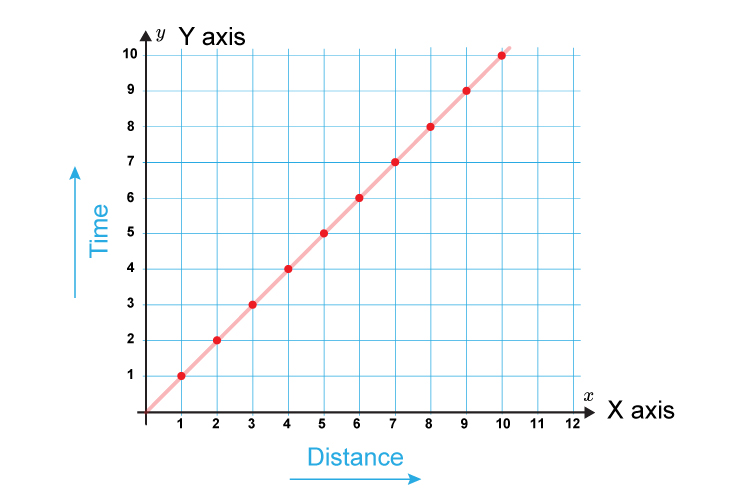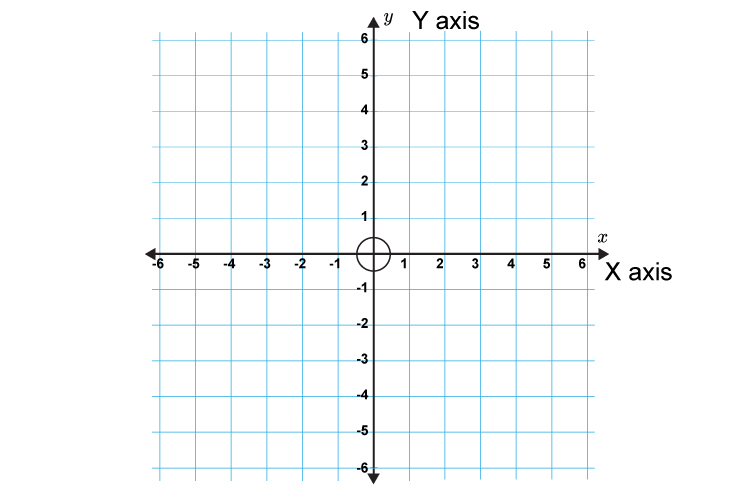# Graphs

## x and y axis

The x axis is the horizontal axis and the y axis is the vertical axis.Would you feel safer balancing the egg on the x or the y? We think you would prefer balancing the egg on X's horizontal expanse.

And another way to remember is:

X is a cross, so the x axis is across.

x is a cross, so the x axis is across. Therefore, the y axis is vertical.

And a further method to remember, for the poets amongst us:

X to the left, Y to the skies.

Example 1All graphs have an x axis and a y axis.

Example 2Both the x axis and y axis of a graph may also show negative values, as in the one above.